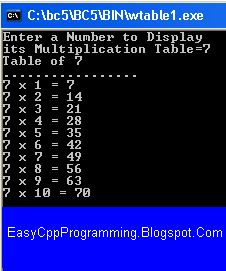# C++ Tutorial With Basic Programs for Beginners

C++ tutorial, C++ programs, C++ code, C++ flowcharts, C++ statements, C++ syntax, C++ examples

## Thursday, January 9, 2014

### Program Multiplication Table by While Loop

Q. Write a C++ Program to input a number and then display its multiplication table.Easyway C++ - Write a C++ Program to input a number and then display its multiplication table -Easyway C++
#include<iostream>
#include<conio.h>

int main()
{
int num;
clrscr();
cout<<"Enter a Number to Display\nits Multiplication Table=";
cin>>num;
cout<<"Table of "<<num<<endl<<"................."<<endl;
int i=1;
while(i<=10)
{
cout<<num<<" x "<<i<<" = "<<num*i<<endl;
i++;
}
getch();
return 0;
}

### Logic of the Multiplication Table Program in C++

1.  First of all, we input a number to display its table in a variable num.
2.  Initialize a loop control variable i to 1.
3. Set a while loop with the condition i<=10 because we wish to repeat loop body for 10 times from i=1 to i=10
4. cout<<num<<" x "<<i<<" = "<<num*i<<endl;
The above statement will display one line of multiplication table as follows
Let the entered number is 7 and i = 1
7 x 1 = 7
5. The statement i++; will cause increment of 1 to i, so that i has the value 2 now.
6.

int i=1;
while(i<=10)
{
cout<<num<<" x "<<i<<" = "<<num*i<<endl;
i++;
}

This while loop will repeat loop body as long as the condition remains true for the value of i = 1 to 10.
When i becomes 11 then the condition i<=10  takes the form 11<=10 which gives false. Hence loop will stop.
Share:

#### Post a Comment

We Love To Hear From You!